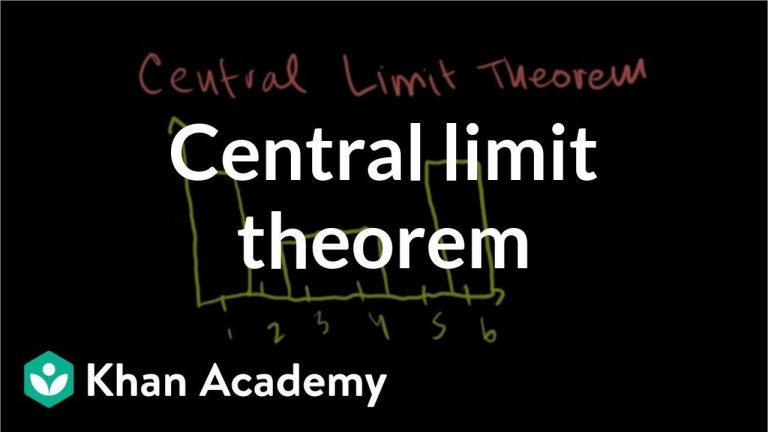November 27, 2023

# The Ultimate Roadmap to Master Central Limit Theorem### Understanding the Central Limit Theorem (CLT) in detail is crucial for a Data Dcientist. Here are the key topics that you should delve into to grasp CLT from the root:

1. Sampling and Random Variables:

• Population and Sample: Understand the concepts of a population (the entire dataset) and a sample (a subset of the population).
• Random Variables: Learn about random variables that represent uncertain quantities in a dataset.

2. Probability Distributions:

• Continuous and Discrete Distributions: Familiarize yourself with different types of probability distributions, like normal, uniform, and binomial distributions.
• Mean and Variance: Understand the concepts of mean (average) and variance (measure of data spread) in probability distributions.

3. Central Limit Theorem Basics:

• The CLT Statement: Comprehend the CLT’s core idea: as sample size increases, the distribution of sample means approaches a normal distribution regardless of the population distribution.

4. Sampling Distribution:

• Sampling Distribution of Sample Mean: Learn about the distribution of the sample means, which becomes normal as per CLT.
• Sampling Distribution Properties: Explore the mean and standard deviation of the sampling distribution of the sample mean.

5. Standard Error and Variability:

• Standard Error of the Mean (SE): Understand SE as the standard deviation of the sample means. Learn its calculation and significance.
• Variance Reduction: Comprehend how larger sample sizes reduce variability in the sample mean distribution.

6. Z-Scores and Standardization:

• Z-Scores: Grasp the concept of Z-scores, which measure how many standard deviations a data point or sample mean is from the population mean.
• Standardizing Data: Learn how to standardize data using Z-scores to make comparisons and calculations easier.

7. Confidence Intervals:

• Confidence Interval Basics: Understand confidence intervals as ranges within which population parameters (like the mean) are likely to fall.
• Calculating Confidence Intervals: Learn to construct confidence intervals using the sample mean, standard error, and critical Z-values.

8. Hypothesis Testing and Z-Tests:

• Hypothesis Testing Framework: Explore hypothesis testing as a process to make decisions about population parameters based on sample data.
• Z-Tests: Learn about Z-tests, which use Z-scores and standard errors to compare sample means with population parameters.

9. Practical Applications and Limitations:

• Real-World Use Cases: Study how data scientists apply CLT to solve problems in various fields.
• Limitations of CLT: Understand situations where CLT may not hold true due to specific population distributions or small sample sizes.

10. Sample Size Considerations:

• Sample Size Requirements: Learn about the recommended sample size (often n ≥ 30) for CLT to work effectively.
• Impact of Sample Size: Understand how larger sample sizes lead to better approximations to normality.

11. Simulation and Experimentation:

• Simulating CLT: Experiment with simulated data and various sample sizes to observe the CLT in action.
• Practical Implementation: Apply CLT principles in Python/R to analyze real datasets and draw inferences.

By delving into these key topics, you’ll develop a deep understanding of the Central Limit Theorem from its fundamental concepts to its practical applications. This comprehensive knowledge will empower you to make informed decisions and draw accurate insights from data using the principles of CLT.